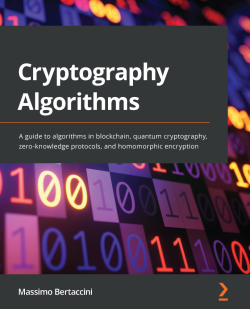# Cryptography Algorithms4 (2 reviews total)
By Massimo Bertaccini
• FREE Subscription Access now
• \$46.99 Print
• \$37.99 eBook
What do you get with a Packt Subscription?

• Constantly updated with 100+ new titles each month
• Breadth and depth in over 1,000+ technologies

Cryptography Algorithms is designed to help you get up and running with modern cryptography algorithms. You'll not only explore old and modern security practices but also discover practical examples of implementing them effectively.

The book starts with an overview of cryptography, exploring key concepts including popular classical symmetric and asymmetric algorithms, protocol standards, and more. You'll also cover everything from building crypto codes to breaking them. In addition to this, the book will help you to understand the difference between various types of digital signatures. As you advance, you will become well-versed with the new-age cryptography algorithms and protocols such as public and private key cryptography, zero-knowledge protocols, elliptic curves, quantum cryptography, and homomorphic encryption. Finally, you'll be able to apply the knowledge you've gained with the help of practical examples and use cases.

By the end of this cryptography book, you will be well-versed with modern cryptography and be able to effectively apply it to security applications.

Publication date:
March 2022
Publisher
Packt
Pages
358
ISBN
9781789617139

# Chapter 1: Deep Diving into Cryptography

This chapter provides an introduction to cryptography, what it is needed for, and why it is so important in IT. This chapter also gives a panoramic view of the principal algorithms from the history of cryptography, from the Caesar algorithm to the Vernam cipher and other lesser-known algorithms, such as the Beale cipher. Then, Rivest-Shamir-Adleman (RSA), Diffie–Hellman, and other famous algorithms will be described in detail in the proceeding part of this book. Finally, this chapter will give you the instruments to learn cryptography and the pillars of security conservation.

In this chapter, we will cover the following topics:

• A brief introduction to cryptography
• Basic definitions and principal mathematical notations used in the book
• Binary conversion and ASCII code
• Fermat Last's Theorem, prime numbers, and modular mathematics
• The history of the principal cryptographic algorithms and an explanation of some of them (Rosetta cipher, Caesar, ROT13, Beale, Vernam)
• Security notation (semantic, provable, OTP, and so on)

# An introduction to cryptography

One of the most important things in cryptography is to understand definitions and notations. I have never been a fan of definitions and notations, first of all, because I am the only one to use notations that I've invented. But I realize that it is very important, especially when we are talking about something related to mathematics, to agree among ourselves. Thus, in this section, I will introduce basic information and citations relating to cryptography.

In mathematics and computer science, an algorithm is a finite sequence of well-defined computer-implementable instructions.

An important question is: what is a cipher?

A cipher is a system of any type able to transform plaintext (a message) into not-intelligible text (a ciphertext or cryptogram):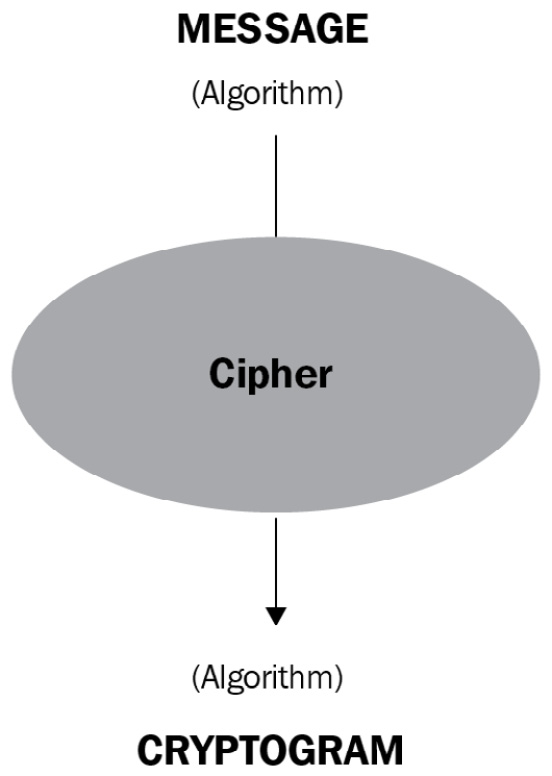Figure 1.1 – Encryption process

To get some utility from a cipher, we have to set up two operations: encryption and decryption. In simpler terms, we have to keep the message secret and safe for a certain period of time.

We define `M` as the set of all the messages and `C` as the set of all the cryptograms.

Encryption is an operation that transforms a generic message, `m`, into a cryptogram, `c`, applying a function, `E`:

`m ------- > f(E) --------- > c `

Decryption is an operation that returns the message in cleartext, `m`, from the cryptogram, `c`, applying a function, `D`:

`C ------- > f(D) --------- > m `

Mathematically, `D(E(M))=` `M`.

This means that the `E` and `D` functions are the inverse of each other, and the `E` function has to be injective. Injective means different `M` values have to correspond to different `C` values.

Note that it doesn't matter whether I use capital letters or lowercase, such as `(M)` or `(m)`; it's inconsequential at the moment. For the moment, I have used round brackets indiscriminately, but later I will use square brackets to distinguish secret elements of a function from known ones, for which I will use square brackets. So, the secret message `M` will be written as `[M]`, just like any other secret parameter. Here, just showing how the algorithms work is within our scope; we'll leave their implementation to engineers.

There is another important notation that is key to encryption/decryption. To encrypt and decrypt a message, it is necessary to set up a key. In cryptography, a key is a parameter that determines the functional output of a cryptographic algorithm or cipher. Without a key, the algorithm would produce no useful results.

We define `K` as the set of all the keys used to encrypt and decrypt `M`, and `k` as the single encryption or decryption key, also called the session key. However, these two ways to define a key (a set of keys is `K` and a single key is `k`) will always be used, specifying what kind of key it is (private or public).

Now that we understand the main concepts of cryptographic notation, it is time to explain the difference between private and public keys:

• In cryptography, a private or secret key (`Kpr`), denoted as `[K]` or `[k]`, is an encryption/decryption parameter known only to one, both, or multiple parties in order to exchange secret messages.
• In cryptography, a public key (`Kpu`) or `(K)` is an encryption key known by everyone who wants to send a secret message or authenticate a user.

So, what is the main difference between private and public keys?

The difference is that a private key is used both to encrypt and/or decrypt a message, while a public key is used only to encrypt a message and verify the identity (digital signatures) of humans and computers. This is a substantial and very important issue because it determines the difference between symmetric and asymmetric encryption.

Let's give a generic definition of these two methods of encryption:

• Symmetric encryption uses only one shared key to both encrypt and decrypt the message.
• Asymmetric encryption implements more parameters to generate a public key (to encrypt the message) and just one private key to decrypt the message.

As we will see later on, private keys are used in symmetric encryption to encrypt/decrypt the message with the same key and in asymmetric encryption in a general way for decryption, whereas public keys are used only in asymmetric encryption to encrypt the message and to perform digital signatures. You will see the function of these two types of keys later, but for now, keep in mind that a private key is used both in symmetric and asymmetric encryption, while a public key is used only for asymmetric encryption. Note that it's not my intention to discuss academic definitions and notation, so please try to figure out the scope and the use of each element.

One of the main problems in cryptography is the transmission of the key, or the key exchange. This problem resulted in strong diatribes in the community of mathematicians and cryptographers because it was very hard to determine how to transmit a key while avoiding physically exchanging it.

For example, if Alice and Bob wanted to exchange a key (before the advent of asymmetric encryption), the only trusted way to do that was to meet physically in one place. This condition caused a lot of problems with the massive adoption of telecommunication systems and the internet. The first problem was that internet communication relies on data exchange over unsafe channels. As you can easily understand, if Alice communicates with Bob through an insecure public communication channel, the private key has a severe possibility of being compromised, which is extremely dangerous for the security and privacy of communications.

For this reason, this question arises: if we use a symmetric cipher to protect our secret information, how can we securely exchange the secret key?

A simple answer is the following: we have to provide a secure channel of communication to exchange the key.

Someone could then reply: how do we provide a secure channel?

We will find the answer, or rather multiple answers, later on in this book. Even in tough military applications, such as the legendary red line between the leaders of the US and USSR during the Cold War, symmetric communication keys were used; nowadays, it is common to use asymmetric encryption to exchange a key. Once the key has been exchanged, the next communication session is combined with symmetric encryption to encrypt the messages transmitted.

For many reasons, asymmetric encryption is a good way to exchange a key and is good for authentication and digital signatures. Computationally, symmetric encryption is better because it can work with lower bit-length keys, saving a lot of bandwidth and timing. So, in general, its algorithms work efficiently for security using keys of 256-512 bits compared to the 4,000+ bits of asymmetric RSA encryption, for example. I will explain in detail why and how that is possible later during the analysis of the algorithms in asymmetric/symmetric encryption.

While in this book I will analyze many kinds of cryptographic techniques, essentially, we can group all the algorithms into two big families: symmetric and asymmetric encryption.

We need some more definitions to understand cryptography well:

• Plaintext: In cryptography, this indicates unencrypted text, or everything that could be exposed in public. For example, `(meet you tomorrow at 10 am)` is plaintext.
• Ciphertext: In cryptography, this indicates the result of the text after having performed the encryption procedure. For example, `meet you tomorrow at 10 am` could become `[x549559*ehebibcm3494]` in ciphertext.

As I mentioned before, I use different brackets to identify plaintext and ciphertext. In particular, these brackets (`…`) identify plaintext, while square brackets `[…]` identify ciphertext.

## Binary numbers, ASCII code, and notations

When we manipulate data with computers, it is common to use data as strings of `0` and `1` named bits. So, numbers can be converted into bits (base 2) rather than into base 10, like our numeric system. Let's just have a look at how the conversion mechanism works. For example, the number (`123`) can be written in base 10 as `1*10^2+2*10^1 +3*10^0`.

Likewise, we can convert a base 10 number to a base 2 number. In this case, we use the example of the number `29`: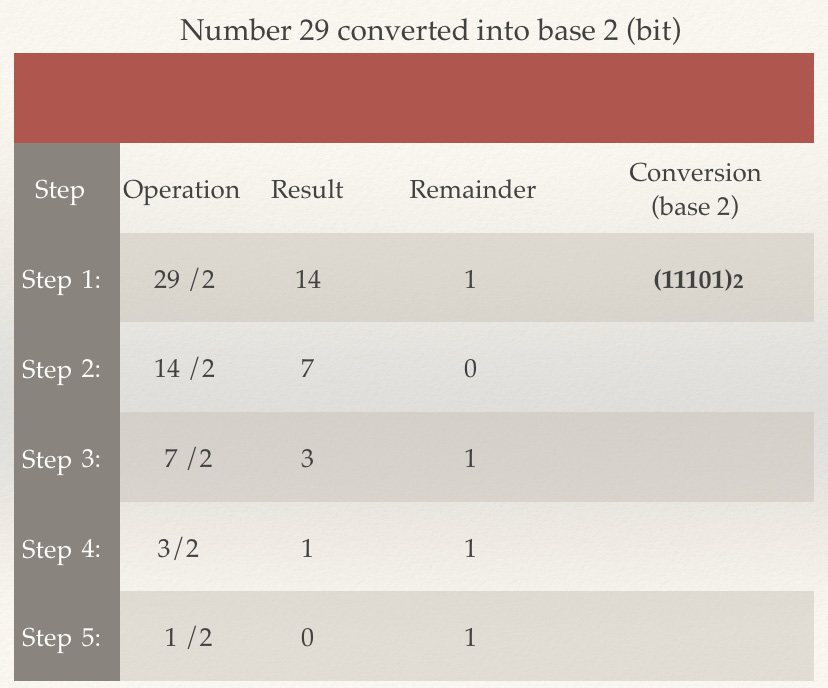Figure 1.2 – Conversion of the number 29 into base 2 (bits)

The remainder of a division is very popular in cryptography because modular mathematics is based on the concept of remainders. We will go deeper into this topic in the next section, when I explain prime numbers and modular mathematics.

To transform letters into a binary system to be encoded by computers, the American Standards Association invented the ASCII code in 1960.

From the ASCII website, we have the following definition:

"ASCII stands for American Standard Code for Information Interchange. It's a 7-bit character code where every single bit represents a unique character."

The following is an example of an ASCII code table with the first 10 characters: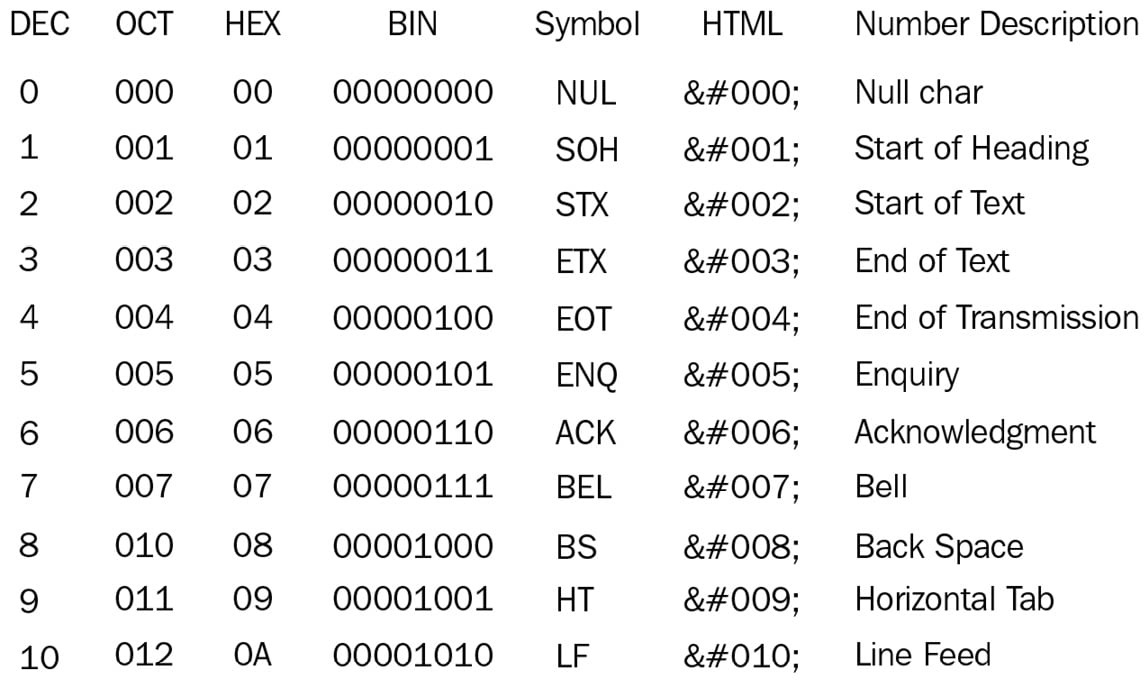Figure 1.3 – The first 10 characters and symbols expressed in ASCII code

Note that I will often use in my implementations, made with the Wolfram Mathematica research software, the character `88` as `X` to denote the message number to encrypt. In ASCII code, the number `88` corresponds to the symbol `X`, as you can see in the following example:

`88    130    58    01011000    X    &#88;    Uppercase X`

You can go to the Appendix section at the end of the book to find all the notation used in this book both for the algorithms and their implementation with Mathematica code.

## Fermat's Last Theorem, prime numbers, and modular mathematics

When we talk about cryptography, we have to always keep in mind that this subject is essentially related to mathematics and logic. Before I start explaining Fermat's Last Theorem, I want to introduce some basic notation that will be used throughout the book to prevent confusion and for a better understanding of the topic. It's important to know that some symbols, such as `=`, `≡` (equivalent), and `:=` (this last one you can find in Mathematica to compute `=`), will be used by me interchangeably. It's just a way to tell you that two elements correspond to each other in equal measure; it doesn't matter whether it is in a finite field (don't worry, you will become familiar with this terminology), computer science, or in regular algebra. Mathematicians may be horrified by this, but I trust your intelligence and that you will look for the substance and not for the uniformity.

Another symbol, `≈` (approximate), can be used to denote similar approximative elements.

You will also encounter the `^` (exponent) symbol in cases such as in a classical way to express exponentiation: ax (`a` elevated to `x`), for example.

The `≠` symbol, as you should remember from high school, means not equal or unequal, which is the same as the meaning of `≢`, that is, not equivalent.

However, you will always get an explanation of the equations, so if you are not very familiar with mathematical and logical notation, you can rely on the descriptions. Anyway, I will explain each case as we come across new notation.

A prime number is an integer that can only be divided by itself and 1, for example, 2, 3, 5, 7….23….67……p.

Prime numbers are the cornerstones of mathematics because all other composite numbers originate from them.

Now, let's see what Fermat's Last Theorem is, where it is applied, and why it is useful for us.

Fermat's Last Theorem is one of the best and most beautiful theorems of classical mathematics strictly related to prime numbers. According to Wikipedia, "In number theory, Fermat's Last Theorem (sometimes called Fermat's conjecture, especially in older texts) states that no three positive integers a, b, and c satisfy the equation a^n + b^n = c^n for any integer value of n greater than 2. The cases n = 1 and n = 2 have been known since antiquity to have infinitely many solutions."

In other words, it tells us that given the following equation, for any exponent, `n>3`, there is no integer, `a`, `b`, or `c`, that verifies the sum:

`a^n+b^n = c^n`

Why is this theorem so important for us? Firstly, it's because Fermat's Last Theorem is strictly related to prime numbers. In fact, given the properties of primes, in order to demonstrate Fermat's Last Theorem, it's sufficient to demonstrate the following:

`a^p+b^p ≠ c^p`

Here, `p` is any prime number greater than 2.

Fermat stated he had a proof that was too large to fit in the margin of his notes.

Fermat himself noted in a paper that he had a beautiful demonstration of the problem, but it has never been found.

Wiles' proof is more than 200 pages long and is immensely difficult to understand. The proof is based on elliptic curves: these curves take a particular form when they are represented in a modular form. Wiles arrived at his conclusion after 7 years and explained his proof at a mathematicians' congress in 1994. I will explain the proof and part of the logic used in Chapter 7, Elliptic Curves. Right now, we just assume that to demonstrate Fermat's Last Theorem, Wiles needed to rely on the Taniyama Shimura conjecture, which states that elliptic curves over the field of rational numbers are related to modular forms. Again, don't worry if this seems too complicated; eventually, as we progress, it will start making sense.

We will deeply analyze Fermat's Last Theorem in Chapter 6, New Algorithms in Public/Private Key Cryptography, when I introduce the MB09 algorithm based on Fermat's Last Theorem, among other innovative algorithms in public/private keys. Moreover, we will analyze the elliptic curves applied in cryptography in Chapter 7, Elliptic Curves.

Fermat was obsessed with prime numbers, just like many other mathematicians; he searched for prime numbers and their properties throughout his life. He tried to attempt to find a general formula to represent all the primes in the universe, but unluckily, Fermat, just like many other mathematicians, only managed to construct a formula for some of them. The following is Fermat's prime numbers formula:

`2^2n + 1    for some positive integer n`

If we substitute `n` with integers, we can obtain some prime numbers:

```n = 1, p = 5
n = 2, p = 17
n = 3, p = 65 (not prime)
n = 4, p = 257```

Probably more famous but very similar is the Mersenne prime numbers formula:

```2^n - 1   for some positive integer n
n = 1, p=1
n = 2, p=3
n = 3, p=7
n = 4, p=15 (not prime)
n = 5, p=31```

Despite countless attempts to find a formula that exclusively represents all prime numbers, nobody has reached this goal as yet.

Great Internet Mersenne Prime Search (GIMPS) is a research project that aims to discover the newest and biggest prime numbers with Mersenne's formula.

If you explore the GIMPS website, you can discover the following:

All exponents below 53 423 543 have been tested and verified.

All exponents below 92 111 363 have been tested at least once.

51st Known Mersenne Prime Found!

December 21, 2018 — The Great Internet Mersenne Prime Search (GIMPS) has discovered the largest known prime number, 2^82,589,933-1, having 24,862,048 digits. A computer volunteered by Patrick Laroche from Ocala, Florida made the find on December 7, 2018. The new prime number, also known as M82589933, is calculated by multiplying together 82,589,933 twos and then subtracting one. It is more than one and a half million digits larger than the previous record prime number.

Besides that, GIMPS is probably the first decentralized example of how to split CPU and computer power to reach a common goal. But why all this interest in finding big primes?

There are at least three answers to this question: the passion for pure research, the money – because there are several prizes for those who find big primes – and finally, because prime numbers are important for cryptography, just like oxygen is for humans. This is also the reason why there is prize money for discovering big prime numbers.

You will understand that most algorithms of the next generation work with prime numbers. But how do you discover whether a number is prime?

In mathematics, there is a substantial computation difference between the operation of multiplication and division. Division is a lot more computationally expensive than multiplication. This means, for instance, that if I compute `2^x`, where `x` is a huge number, it is easy to operate the power elevation but is extremely difficult to find the divisors of that number.

Because of this, mathematicians such as Fermat struggled to find algorithms to make this computation easier.

In the field of prime numbers, Fermat produced another very interesting theorem, known as Fermat's Last Theorem. Before explaining this theorem, it is time to understand what modular arithmetics is and how to compute with it.

The simplest way to learn modular arithmetics is to think of a clock. When we say: "Hey, we can meet at 1 p.m." actually we calculate that 1 is the first hour after 12 (the clock finishes its circular wrap).

So, we can say that we are unconsciously calculating in modulus 12 written by the notation (`mod 12`), where integers wrap around when reaching a certain value (in this case 12), called the modulus.

Technically, the result of a calculation with a modulus consists of the remainder of the division between the number and the modulus.

For example, in our clock, we have the following:

`13 ≡ 1 (mod 12) `

This means that `13` is congruent to `1` in modulus `12`. You can consider congruent to mean equal. In other words, we can say that the remainder of the division of `13:12` is `1`: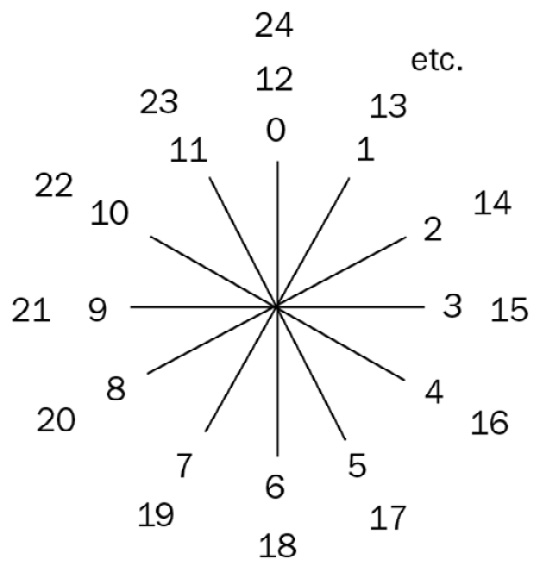Figure 1.4 – Example of modular arithmetics with a clock

Fermat's Last Theorem states that if `(p)` is a prime number, then for any integer `(a)` elevated to the prime number `(p)` we find `(a)` as the result of the following equation:

`a^p ≡ a (mod p)`

For example, if `a = 2` and `p = 3`, then `2^3 = 2 (mod 3)`. In other terms, we find the rest of the division `8 : 3 = 2` with reminder `2`.

Fermat's Last Theorem is the basis of the Fermat primality test and is one of the fundamental parts of elementary number theory.

Fermat's Last Theorem states that a number, `p`, is probably prime in the following instance:

`a^p ≡ a (mod p)`

Now that we have refreshed our knowledge on the operations of bit conversion, we have seen what ASCII code looks like, and we have explored the basic notation of mathematics and logic, we can start our journey into cryptography.

# A brief history and a panoramic overview of cryptographic algorithms

Nobody probably knows which cryptogram was the first to be invented. Cryptography has been used for a long time, approximately 4,000 years, and it has changed its paradigms a lot. First, it was a kind of hidden language, then cryptography was based on a transposition of letters in a mechanical fashion, then finally, mathematics and logic were used to solve complicated problems. What will the future hold? Probably, new methods will be invented to hide our secrets: quantum cryptography, for example, is already being experimented with and will come soon. I will explain new algorithms and methods throughout this book, but let me use this section to show you some interesting ciphers related to the classical period. Despite the computation power we have now, some of these algorithms have not yet been broken.

## Rosetta Stone

One of the first extraordinary examples of cryptography was hieroglyphics. Cryptography means hidden words and comes from the union of two Greek words: κρυπτός (crypto) and γράφω (graphy). Among the many definitions of this word, we find the following: converting ordinary plaintext into unintelligible text and vice versa. So, we can include hieroglyphics in this definition, because we discovered how to re-convert their hidden meaning into intelligible text only after the Rosetta Stone was found. As you will probably remember from elementary school, the Rosetta Stone was written in three different languages: Ancient Egyptian (using hieroglyphics), Demotic, and Ancient Greek. The Rosetta Stone could only be decrypted because Ancient Greek was well known at the time: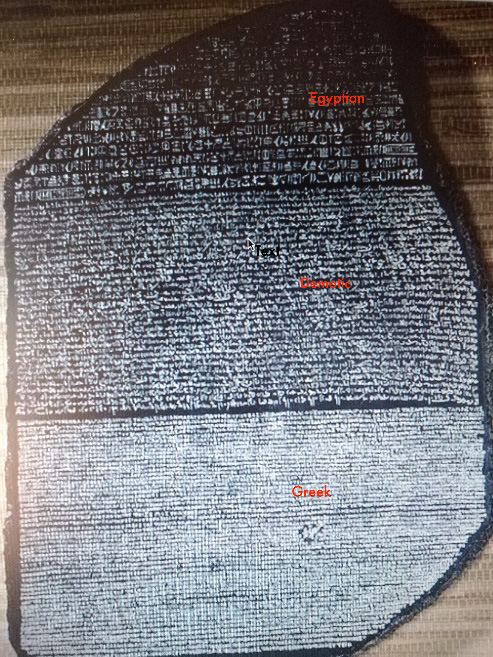Figure 1.5 – Rosetta Stone with the three languages detected

Hieroglyphics were a form of communication between the people of a country. The same problem of deciphering an unknown language could occur in the future if and when we get in contact with an alien population. A project called SETI (https://www.seti.org/) focuses on this: "From microbes to alien intelligence, the SETI Institute is America's only organization wholly dedicated to searching for life in the universe." Maybe if one day we get in contact with alien creatures, we will eventually understand their language. You can imagine that hieroglyphics (at the time) appeared as impenetrable as an alien language for someone who had never encountered this form of communication.

## Caesar cipher

Continuing our journey through history, we find that during the Roman Empire, cryptography was used to transmit messages from the generals to the commanders and to soldiers. In fact, we find the famous Caesar cipher. Why is this encrypting method so famous in the history of cryptography?

This is not only because it was used by Caesar, who was one of the most valorous Roman statesmen/generals, but also because this method was probably the first that implemented mathematics.

This cipher is widely known as a shift cipher. The technique of shifting is very simple: just shift each letter you want to encrypt a fixed number of places in the alphabet so that the final effect will be to obtain a substitution of each letter for another one. So, for example, if I decide to shift by three letters, then A will become D, E becomes H, and so on.

For example, in this case, by shifting each letter three places, implicitly we have created a secret cryptographic key of `[K=3]`: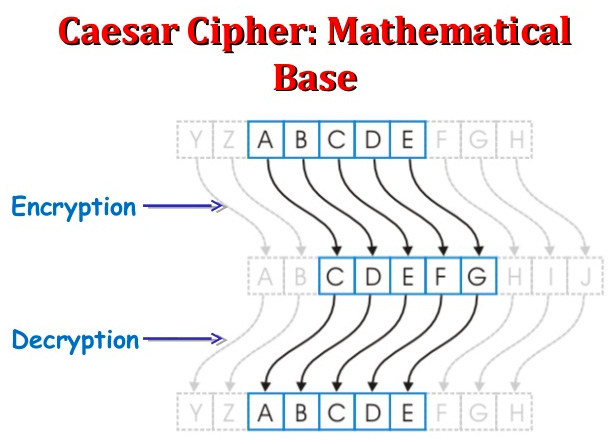Figure 1.6 – The transposition of the letters in the Caesar cipher during the encryption and decryption processes

It is obviously a symmetric key encryption method. In this case, the algorithm works in the following way:

• Use this key: `(+3)`.
• Message: `HELLO`.
• To encrypt: Take every letter and shift by `+3` steps.
• To decrypt: Take every letter and de-shift by `-3`.

You can see in the following figure how the process of encryption and decryption of the Caesar algorithm works using `key = +3`; as you'll notice, the word `HELLO` becomes `KHOOR` after encryption, and then it returns to `HELLO` after decryption: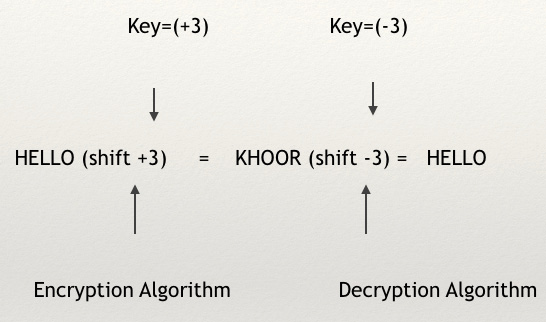Figure 1.7 – Encryption and decryption using Caesar's algorithm

As you can imagine, the Caesar algorithm is very easy to break with a normal computer if we set a fixed key, as in the preceding example. The scheme is very simple, which, for a cryptographic algorithm, is not a problem. However, the main problem is the extreme linearity of the underlying mathematics. Using a brute-force method, that is, a test that tries all the combinations to discover the key after having guessed the algorithm used (in this case, the shift cipher), we can easily break the code. We have to check at most 25 combinations: all the letters of the English alphabet (26) minus one (that is, the same intelligible plaintext form). This is nothing compared to the billions and billions of attempts that a computer has to make in order to break a modern cryptographic algorithm.

However, there is a more complex version of this algorithm that enormously increases the efficiency of the encryption.

If I change the key for each letter and I use that key to substitute the letters and generate the ciphertext, then things become very interesting.

Let's see what happens if we encrypt `HELLO` using a method like this:

1. Write out the alphabet.
2. Choose a passphrase (also known as a keyphrase) such as `[JULIUSCAESAR]` and repeat it, putting each letter of the alphabet in correspondence with a character from the passphrase in the second row, as shown in the following screenshot.
3. After we have defined the message to encrypt, for each character composing the message (in the first row) select the corresponding character of the keyphrase (in the second row).
4. Pick up the selected corresponding characters in the second row to create the ciphertext.

Finding it a little bit complicated? Don't worry, the following example will clarify everything.

Let's encrypt `HELLO` with the keyphrase `[JULIUSCAESARJULIUS…]`: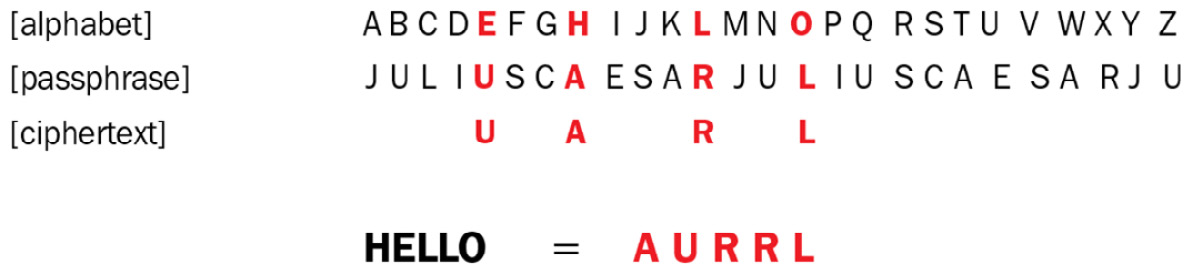Figure 1.8 – Encrypting HELLO with a keyphrase becomes harder to attack

Thus, encrypting the plaintext `HELLO` using the alphabet and a key (or better, a passphrase or a keyphrase), `JULIUSCAESAR`, repeated without any spaces, we obtain the correspondent ciphertext: `AURRL`.

So, `H` becomes `A`, `E` becomes `U`, `L` becomes `R` (twice), and `O` becomes `L`.

Earlier, we only had to check 25 combinations to find the key in the Caesar cipher; here, things have changed a little bit, and there are 26 possibilities to discover the key! That means multiply 1*2*3...*26, which results in 403,291,461,126,605,635,584,000,000. This is a very big number. In fact, it is about one-third of all the atoms in the universe. Computationally, it is pretty hard to discover the key, even for a modern computer using a brute-force method.

Another advantage of building a cryptogram like this is that it is easy to memorize the keyword or keyphrase and hence work out the ciphertext. But let's see a cipher that is performed with a similar technique and is used in commercial contexts.

## ROT13

A modern example of an algorithm that is used on the internet is ROT13. Essentially, this is a simple cipher derived from the Caesar cipher with a shift of `(+13)`. Computationally, it is easy to break the Caesar cipher, but it yields an interesting effect: if we shift to the left or to the right, we will have the same result.

Just like the preceding example, in ROT13, we have to select letters that correspond to the pre-selected key. Essentially, the difference here is that instead of applying a keyphrase to perform the ciphertext, we will use 13 letters from the English alphabet as the key generator. ROT13 takes in encryption only the letters that occur in the English alphabet and not numbers, symbols, or other characters, which are left as they are. The ROT13 function essentially encrypts the plaintext with a key determined by the first 13 letters transposed into the second 13 letters, and the inverse for the second 13 letters.

Take a look at the following example to better understand the encryption scheme: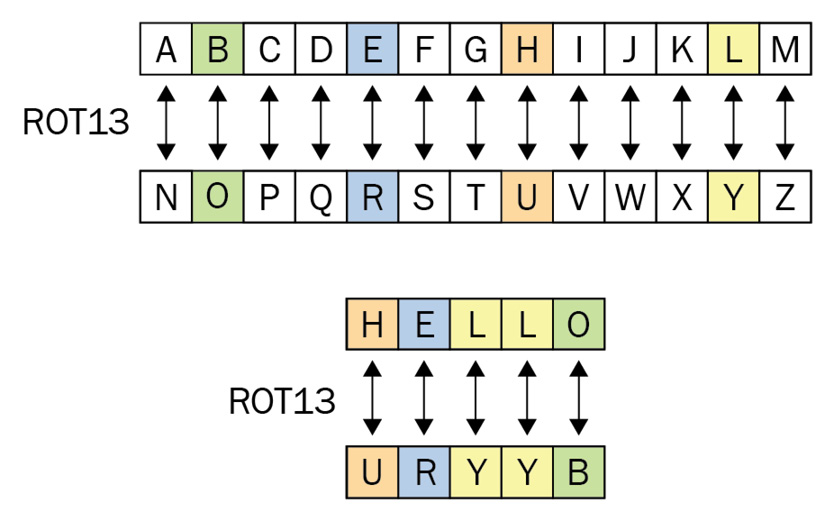Figure 1.9 – The encryption scheme in ROT13

As you can see in the preceding diagram, `H` becomes `U`, `E` becomes `R`, `L` becomes `Y` (twice), and `O` becomes `B`:

`HELLO = URYYB`

The key consists of the first 13 letters of the alphabet up to `M`, which becomes `Z`, then the sequence wraps back to `N`, which becomes `A`, `O` becomes `B`, and so on to `Z`, which becomes `M`.

ROT13 was used to hide potentially offensive jokes or obscure an answer in the `net.jokes` newsgroup in the early 1980s.

Also, even though ROT13 is not intended to be used for a high degree of secrecy, it is still used in some cases to hide email addresses from unsophisticated spambots. ROT13 is also used for the scope of circumventing spam filters such as obscuring email content. This last function is not recommended because of the extreme vulnerability of this algorithm.

However, ROT13 was used by Netscape Communicator – the browser organization that released https://www.mozilla.org – to store email passwords. Moreover, ROT13 is used in Windows XP to hide some registry keys, so you can understand how sometimes even big corporations can have a lack of security and privacy in communications.

## The Beale cipher

Going back to the history of cryptography, I would like to show you an amazing method of encryption whose cipher has not been decrypted yet, despite the immense computational power of our modern calculators. Very often, cryptography is used to hide precious information or fascinating treasure, just as in the mysterious story that lies behind the Beale cipher.

In order to better understand the method of encryption adopted in this cipher, I think it is interesting to know the story (or legend) of Beale and his treasure.

The story involves buried treasure with a value of more than \$20 million, a mysterious set of encrypted documents, Wild West cowboys, and a hotel owner who dedicated his life to struggling with the decryption of these papers. The whole story is contained in a pamphlet that was published in 1885.

The story (you can find the whole version here: http://www.unmuseum.org/bealepap.htm) begins in January 1820 in Lynchburg, Virginia at the Washington Hotel where a man named Thomas J. Beale checked in. The owner of the hotel, Robert Morriss, and Beale became friends, and because Mr. Morriss was considered a trustworthy man, he received a box containing three mysterious papers covered in numbers.

After countless troubles and many years of struggle, only the second of the three encrypted papers was deciphered.

What exactly does Beale's cipher look like?

The following content consists of three pages, containing only numbers, in an apparently random order.

The first paper is as follows:

`71, 194, 38, 1701, 89, 76, 11, 83, 1629, 48, 94, 63, 132, 16, 111, 95, 84, 341, 975, 14, 40, 64, 27, 81, 139, 213, 63, 90, 1120, 8, 15, 3, 126, 2018, 40, 74, 758, 485, 604, 230, 436, 664, 582, 150, 251, 284, 308, 231, 124, 211, 486, 225, 401, 370, 11, 101, 305, 139, 189, 17, 33, 88, 208, 193, 145, 1, 94, 73, 416, 918, 263, 28, 500, 538, 356, 117, 136, 219, 27, 176, 130, 10, 460, 25, 485, 18, 436, 65, 84, 200, 283, 118, 320, 138, 36, 416, 280, 15, 71, 224, 961, 44, 16, 401, 39, 88, 61, 304, 12, 21, 24, 283, 134, 92, 63, 246, 486, 682, 7, 219, 184, 360, 780, 18, 64, 463, 474, 131, 160, 79, 73, 440, 95, 18, 64, 581, 34, 69, 128, 367, 460, 17, 81, 12, 103, 820, 62, 116, 97, 103, 862, 70, 60, 1317, 471, 540, 208, 121, 890, 346, 36, 150, 59, 568, 614, 13, 120, 63, 219, 812, 2160, 1780, 99, 35, 18, 21, 136, 872, 15, 28, 170, 88, 4, 30, 44, 112, 18, 147, 436, 195, 320, 37, 122, 113, 6, 140, 8, 120, 305, 42, 58, 461, 44, 106, 301, 13, 408, 680, 93, 86, 116, 530, 82, 568, 9, 102, 38, 416, 89, 71, 216, 728, 965, 818, 2, 38, 121, 195, 14, 326, 148, 234, 18, 55, 131, 234, 361, 824, 5, 81, 623, 48, 961, 19, 26, 33, 10, 1101, 365, 92, 88, 181, 275, 346, 201, 206, 86, 36, 219, 324, 829, 840, 64, 326, 19, 48, 122, 85, 216, 284, 919, 861, 326, 985, 233, 64, 68, 232, 431, 960, 50, 29, 81, 216, 321, 603, 14, 612, 81, 360, 36, 51, 62, 194, 78, 60, 200, 314, 676, 112, 4, 28, 18, 61, 136, 247, 819, 921, 1060, 464, 895, 10, 6, 66, 119, 38, 41, 49, 602, 423, 962, 302, 294, 875, 78, 14, 23, 111, 109, 62, 31, 501, 823, 216, 280, 34, 24, 150, 1000, 162, 286, 19, 21, 17, 340, 19, 242, 31, 86, 234, 140, 607, 115, 33, 191, 67, 104, 86, 52, 88, 16, 80, 121, 67, 95, 122, 216, 548, 96, 11, 201, 77, 364, 218, 65, 667, 890, 236, 154, 211, 10, 98, 34, 119, 56, 216, 119, 71, 218, 1164, 1496, 1817, 51, 39, 210, 36, 3, 19, 540, 232, 22, 141, 617, 84, 290, 80, 46, 207, 411, 150, 29, 38, 46, 172, 85, 194, 39, 261, 543, 897, 624, 18, 212, 416, 127, 931, 19, 4, 63, 96, 12, 101, 418, 16, 140, 230, 460, 538, 19, 27, 88, 612, 1431, 90, 716, 275, 74, 83, 11, 426, 89, 72, 84, 1300, 1706, 814, 221, 132, 40, 102, 34, 868, 975, 1101, 84, 16, 79, 23, 16, 81, 122, 324, 403, 912, 227, 936, 447, 55, 86, 34, 43, 212, 107, 96, 314, 264, 1065, 323, 428, 601, 203, 124, 95, 216, 814, 2906, 654, 820, 2, 301, 112, 176, 213, 71, 87, 96, 202, 35, 10, 2, 41, 17, 84, 221, 736, 820, 214, 11, 60, 760`

The second paper (which was decrypted) is as follows:

`115, 73, 24, 807, 37, 52, 49, 17, 31, 62, 647, 22, 7, 15, 140, 47, 29, 107, 79, 84, 56, 239, 10, 26, 811, 5, 196, 308, 85, 52, 160, 136, 59, 211, 36, 9, 46, 316, 554, 122, 106, 95, 53, 58, 2, 42, 7, 35, 122, 53, 31, 82, 77, 250, 196, 56, 96, 118, 71, 140, 287, 28, 353, 37, 1005, 65, 147, 807, 24, 3, 8, 12, 47, 43, 59, 807, 45, 316, 101, 41, 78, 154, 1005, 122, 138, 191, 16, 77, 49, 102, 57, 72, 34, 73, 85, 35, 371, 59, 196, 81, 92, 191, 106, 273, 60, 394, 620, 270, 220, 106, 388, 287, 63, 3, 6, 191, 122, 43, 234, 400, 106, 290, 314, 47, 48, 81, 96, 26, 115, 92, 158, 191, 110, 77, 85, 197, 46, 10, 113, 140, 353, 48, 120, 106, 2, 607, 61, 420, 811, 29, 125, 14, 20, 37, 105, 28, 248, 16, 159, 7, 35, 19, 301, 125, 110, 486, 287, 98, 117, 511, 62, 51, 220, 37, 113, 140, 807, 138, 540, 8, 44, 287, 388, 117, 18, 79, 344, 34, 20, 59, 511, 548, 107, 603, 220, 7, 66, 154, 41, 20, 50, 6, 575, 122, 154, 248, 110, 61, 52, 33, 30, 5, 38, 8, 14, 84, 57, 540, 217, 115, 71, 29, 84, 63, 43, 131, 29, 138, 47, 73, 239, 540, 52, 53, 79, 118, 51, 44, 63, 196, 12, 239, 112, 3, 49, 79, 353, 105, 56, 371, 557, 211, 505, 125, 360, 133, 143, 101, 15, 284, 540, 252, 14, 205, 140, 344, 26, 811, 138, 115, 48, 73, 34, 205, 316, 607, 63, 220, 7, 52, 150, 44, 52, 16, 40, 37, 158, 807, 37, 121, 12, 95, 10, 15, 35, 12, 131, 62, 115, 102, 807, 49, 53, 135, 138, 30, 31, 62, 67, 41, 85, 63, 10, 106, 807, 138, 8, 113, 20, 32, 33, 37, 353, 287, 140, 47, 85, 50, 37, 49, 47, 64, 6, 7, 71, 33, 4, 43, 47, 63, 1, 27, 600, 208, 230, 15, 191, 246, 85, 94, 511, 2, 270, 20, 39, 7, 33, 44, 22, 40, 7, 10, 3, 811, 106, 44, 486, 230, 353, 211, 200, 31, 10, 38, 140, 297, 61, 603, 320, 302, 666, 287, 2, 44, 33, 32, 511, 548, 10, 6, 250, 557, 246, 53, 37, 52, 83, 47, 320, 38, 33, 807, 7, 44, 30, 31, 250, 10, 15, 35, 106, 160, 113, 31, 102, 406, 230, 540, 320, 29, 66, 33, 101, 807, 138, 301, 316, 353, 320, 220, 37, 52, 28, 540, 320, 33, 8, 48, 107, 50, 811, 7, 2, 113, 73, 16, 125, 11, 110, 67, 102, 807, 33, 59, 81, 158, 38, 43, 581, 138, 19, 85, 400, 38, 43, 77, 14, 27, 8, 47, 138, 63, 140, 44, 35, 22, 177, 106, 250, 314, 217, 2, 10, 7, 1005, 4, 20, 25, 44, 48, 7, 26, 46, 110, 230, 807, 191, 34, 112, 147, 44, 110, 121, 125, 96, 41, 51, 50, 140, 56, 47, 152, 540, 63, 807, 28, 42, 250, 138, 582, 98, 643, 32, 107, 140, 112, 26, 85, 138, 540, 53, 20, 125, 371, 38, 36, 10, 52, 118, 136, 102, 420, 150, 112, 71, 14, 20, 7, 24, 18, 12, 807, 37, 67, 110, 62, 33, 21, 95, 220, 511, 102, 811, 30, 83, 84, 305, 620, 15, 2, 10, 8, 220, 106, 353, 105, 106, 60, 275, 72, 8, 50, 205, 185, 112, 125, 540, 65, 106, 807, 138, 96, 110, 16, 73, 33, 807, 150, 409, 400, 50, 154, 285, 96, 106, 316, 270, 205, 101, 811, 400, 8, 44, 37, 52, 40, 241, 34, 205, 38, 16, 46, 47, 85, 24, 44, 15, 64, 73, 138, 807, 85, 78, 110, 33, 420, 505, 53, 37, 38, 22, 31, 10, 110, 106, 101, 140, 15, 38, 3, 5, 44, 7, 98, 287, 135, 150, 96, 33, 84, 125, 807, 191, 96, 511, 118, 40, 370, 643, 466, 106, 41, 107, 603, 220, 275, 30, 150, 105, 49, 53, 287, 250, 208, 134, 7, 53, 12, 47, 85, 63, 138, 110, 21, 112, 140, 485, 486, 505, 14, 73, 84, 575, 1005, 150, 200, 16, 42, 5, 4, 25, 42, 8, 16, 811, 125, 160, 32, 205, 603, 807, 81, 96, 405, 41, 600, 136, 14, 20, 28, 26, 353, 302, 246, 8, 131, 160, 140, 84, 440, 42, 16, 811, 40, 67, 101, 102, 194, 138, 205, 51, 63, 241, 540, 122, 8, 10, 63, 140, 47, 48, 140, 288`

The third paper is as follows:

`317, 8, 92, 73, 112, 89, 67, 318, 28, 96,107, 41, 631, 78, 146, 397, 118, 98, 114, 246, 348, 116, 74, 88, 12, 65, 32, 14, 81, 19, 76, 121, 216, 85, 33, 66, 15, 108, 68, 77, 43, 24, 122, 96, 117, 36, 211, 301, 15, 44, 11, 46, 89, 18, 136, 68, 317, 28, 90, 82, 304, 71, 43, 221, 198, 176, 310, 319, 81, 99, 264, 380, 56, 37, 319, 2, 44, 53, 28, 44, 75, 98, 102, 37, 85, 107, 117, 64, 88, 136, 48, 151, 99, 175, 89, 315, 326, 78, 96, 214, 218, 311, 43, 89, 51, 90, 75, 128, 96, 33, 28, 103, 84, 65, 26, 41, 246, 84, 270, 98, 116, 32, 59, 74, 66, 69, 240, 15, 8, 121, 20, 77, 89, 31, 11, 106, 81, 191, 224, 328, 18, 75, 52, 82, 117, 201, 39, 23, 217, 27, 21, 84, 35, 54, 109, 128, 49, 77, 88, 1, 81, 217, 64, 55, 83, 116, 251, 269, 311, 96, 54, 32, 120, 18, 132, 102, 219, 211, 84, 150, 219, 275, 312, 64, 10, 106, 87, 75, 47, 21, 29, 37, 81, 44, 18, 126, 115, 132, 160, 181, 203, 76, 81, 299, 314, 337, 351, 96, 11, 28, 97, 318, 238, 106, 24, 93, 3, 19, 17, 26, 60, 73, 88, 14, 126, 138, 234, 286, 297, 321, 365, 264, 19, 22, 84, 56, 107, 98, 123, 111, 214, 136, 7, 33, 45, 40, 13, 28, 46, 42, 107, 196, 227, 344, 198, 203, 247, 116, 19, 8, 212, 230, 31, 6, 328, 65, 48, 52, 59, 41, 122, 33, 117, 11, 18, 25, 71, 36, 45, 83, 76, 89, 92, 31, 65, 70, 83, 96, 27, 33, 44, 50, 61, 24, 112, 136, 149, 176, 180, 194, 143, 171, 205, 296, 87, 12, 44, 51, 89, 98, 34, 41, 208, 173, 66, 9, 35, 16, 95, 8, 113, 175, 90, 56, 203, 19, 177, 183, 206, 157, 200, 218, 260, 291, 305, 618, 951, 320, 18, 124, 78, 65, 19, 32, 124, 48, 53, 57, 84, 96, 207, 244, 66, 82, 119, 71, 11, 86, 77, 213, 54, 82, 316, 245, 303, 86, 97, 106, 212, 18, 37, 15, 81, 89, 16, 7, 81, 39, 96, 14, 43, 216, 118, 29, 55, 109, 136, 172, 213, 64, 8, 227, 304, 611, 221, 364, 819, 375, 128, 296, 1, 18, 53, 76, 10, 15, 23, 19, 71, 84, 120, 134, 66, 73, 89, 96, 230, 48, 77, 26, 101, 127, 936, 218, 439, 178, 171, 61, 226, 313, 215, 102, 18, 167, 262, 114, 218, 66, 59, 48, 27, 19, 13, 82, 48, 162, 119, 34, 127, 139, 34, 128, 129, 74, 63, 120, 11, 54, 61, 73, 92, 180, 66, 75, 101, 124, 265, 89, 96, 126, 274, 896, 917, 434, 461, 235, 890, 312, 413, 328, 381, 96, 105, 217, 66, 118, 22, 77, 64, 42, 12, 7, 55, 24, 83, 67, 97, 109, 121, 135, 181, 203, 219, 228, 256, 21, 34, 77, 319, 374, 382, 675, 684, 717, 864, 203, 4, 18, 92, 16, 63, 82, 22, 46, 55, 69, 74, 112, 134, 186, 175, 119, 213, 416, 312, 343, 264, 119, 186, 218, 343, 417, 845, 951, 124, 209, 49, 617, 856, 924, 936, 72, 19, 28, 11, 35, 42, 40, 66, 85, 94, 112, 65, 82, 115, 119, 236, 244, 186, 172, 112, 85, 6, 56, 38, 44, 85, 72, 32, 47, 63, 96, 124, 217, 314, 319, 221, 644, 817, 821, 934, 922, 416, 975, 10, 22, 18, 46, 137, 181, 101, 39, 86, 103, 116, 138, 164, 212, 218, 296, 815, 380, 412, 460, 495, 675, 820, 952`

The second cipher was successfully decrypted around 1885. Here, I will discuss the main considerations about this kind of cipher.

Since the numbers in the cipher far exceed the number of letters in the alphabet, we can assume that it is not a substitution nor a transposition cipher. So, we can assume that each number represents a letter, but this letter is obtained from a word contained in an external text. A cipher following this criterion is called a book cipher: in the case of a book cipher, a book or any other text could be used as a key. Now, the effective key here is the method of obtaining the letters from the text.

Using this system, the second cipher was decrypted by drawing on the United States Declaration of Independence. Assigning a number to each word of the referring text (the United States Declaration of Independence) and picking up the first letter of each word selected in the key (the list of the numbers, in this case, referred to the second cipher), we can extrapolate the plaintext. The extremely intelligent trick of this cipher is that the key text (the United States Declaration of Independence) is public but at the same time it was unknown to the entire world except for whom the message was intended. Only when someone holds the key (the list of the numbers) and the "key text" can they easily decrypt the message.

Let's look at the process of decrypting the second cipher:

1. Assign to each word of the text a number in order from the first to the last word.
2. Extrapolate the first letter of each word using the numbers contained in the cipher.

The following is the first part of the United States Declaration of Independence (until the 115th word) showing each word with its corresponding number:

`When(1) in(2) the(3) course(4) of(5) human(6) events(7) it(8) becomes(9) necessary(10) for(11) one(12) people(13) to(14) dissolve(15) the(16) political(17) bands(18) which(19) have(20) connected(21) them(22) with(23) another(24) and(25) to(26) assume(27) among(28) the(29) powers(30) of(31) the(32) earth(33) the(34) separate(35) and(36) equal(37) station(38) to(39) which(40) the(41) laws(42) of(43) nature(44) and(45) of(46) nature's(47) god(48) entitle(49) them(50) a(51) decent(52) respect(53) to(54) the(55) opinions(56) of(57) mankind(58) requires(59) that(60) they(61) should(62) declare(63) the(64) causes(65) which(66) impel(67) them(68) to(69) the(70) separation(71) we(72) hold(73) these(74) truths(75) to(76) be(77) self(78) evident(79) that(80) all(81) men(82) are(83) created(84) equal(85) that(86) they(87) are(88) endowed(89) by(90) their(91) creator(92) with(93) certain(94) unalienable(95) rights(96) that(97) among(98) these(99) are(100) life(101) liberty(102) and(103) the(104) pursuit(105) of(106) happiness(107) that(108) to(109) secure(110) these(111) rights(112) governments(113) are(114) instituted(115) ...`

The following numbers represent the first rows of the second cipher; as you can see, the bold words (with their corresponding numbers) correspond to the numbers we find in the ciphertext:

`115, 73, 24, 807, 37, 52, 49, 17, 31, 62, 647, 22, 7, 15, 140, 47, 29, 107, 79, 84, 56, 239, 10, 26, 811, 5, 196, 308, 85, 52, 160, 136, 59, 211, 36, 9, 46, 316, 554, 122, 106, 95, 53, 58, 2, 42, 7, 35...`

The following is the result of decryption using the cipher combined with the key text (the United States Declaration of Independence), picking up the first letter of each corresponding word, that is, the plaintext:

```115 = instituted = I
73 = hold = h
24 = another = a
807 (missing) = v
37 = equal = e
52 = decent = d
49 = entitle = e```

And so on…

I haven't included the entire United States Declaration of Independence; these are only the first 115 words. But if you want, you can visit http://www.unmuseum.org/bealepap.htm and try the exercise to rebuild the entire plaintext.

Here (with some missing letters) is the reconstruction of the first sentence:

`I have deposited in the county of Bedford…….`

If we carry on and compare the numbers with the corresponding numbers of the initial letters of the United States Declaration of Independence, the decryption will be as follows:

I have deposited in the county of Bedford, about four miles from Buford's, in an excavation or vault, six feet below the surface of the ground, the following articles, belonging jointly to the parties whose names are given in number "3," herewith:

The first deposit consisted of one thousand and fourteen pounds of gold, and three thousand eight hundred and twelve pounds of silver, deposited November, 1819. The second was made December, 1821, and consisted of nineteen hundred and seven pounds of gold, and twelve hundred and eighty-eight pounds of silver; also jewels, obtained in St. Louis in exchange for silver to save transportation, and valued at \$13,000.

The above is securely packed in iron pots, with iron covers. The vault is roughly lined with stone, and the vessels rest on solid stone, and are covered with others. Paper number "1" describes the exact locality of the vault so that no difficulty will be had in finding it.

Many other cryptographers and cryptologists have tried to decrypt the first and third Beale ciphers in vain. Others, such as the treasure hunter Mel Fisher, who discovered hundreds of millions of dollars' worth of valuables under the sea, went to Bedford to search the area in order to find the treasure, without success.

Maybe Beale's tale is just a legend. Or maybe it is true, but nobody will ever know where the treasure is because nobody will decrypt the first cipher. Or, the treasure will never be unearthed because someone has already found it.

Anyway, what is really interesting in this story is the implementation of such a strong cipher without the help of any computers or electronic machines; it was just made with brainpower, a pen, and a sheet of paper.

Paradoxically, the number of attempts required to crack the cipher go from 1 to infinity assuming that the attacker works with brute force, exploring all the texts written in the world at that moment. On top of that, what happens if a key text is not public but was written by the transmitter himself and has been kept secret? In this case, if the cryptologist doesn't have the key (so doesn't hold the key text), the likelihood of them decrypting the cipher is zero.

The Beale cipher is also interesting because this kind of algorithm could have new applications in modern cryptography or in the future. Some of these applications could be related to methods of research for encrypted data in cloud computing.

## The Vernam cipher

The Vernam cipher has the highest degree of security for a cipher, as it is theoretically completely secure. Since it uses a truly random key of the same length as the plaintext, it is called the perfect cipher. It's just a matter of entropy and randomness based on Shannon's principle of information entropy that determines an equal probability of each bit contained in the ciphertext. We will revisit this algorithm in Chapter 8, Quantum Cryptography, where we talk about quantum key distribution and the related method to encrypt the plaintext after determining the quantum key. Another interesting implementation is Hyper Crypto Satellite, which uses this algorithm to encrypt the plaintext crafted by a random key transmitted by a satellite radiocommunication and expressed as an infinite string of bits.

But for now, let's go on to explore the main characteristics of this algorithm.

The essential element of the algorithm is using the key only once per session. This feature makes the algorithm invulnerable to attacks against the ciphertext and even in the unlikely event that the key is stolen, it would be changed at the time of the next transmission.

The method is very simple: by adding the key to the message (`mod` `2`) bit by bit, we will obtain the ciphertext. We will see this method, called `XOR`, many times throughout this book, especially when we discuss symmetric encryption in Chapter 2, Introduction to Symmetric Encryption. Just remember that the key has to be of the same length as the message.

A numerical example is as follows:

• `00101001` (plaintext)
• `10101100` (key): Adding each bit (`mod` `2`)
• `10000101` (ciphertext)

Step 1: Transform the plaintext into a string of bits using ASCII code.

Step 2: Generate a random key of the same length as the plaintext.

Step 3: Encrypt by adding modulo 2 (`XOR`) of the plaintext bitwise to the key and obtain the ciphertext.

Step 4: Decrypt by making the inverse operation of adding the ciphertext to the key and obtain the plaintext again.

To make an example with numbers and letters, we will go back to `HELLO`. Let's assume that each letter corresponds to a number, starting from `0 = A`,` 1 = B`,` 2 = C`,` 3 = D`,` 4 = E` … and so on until `25 = Z`.

The random key is `[DGHBC]`.

The encryption will present the following transposition: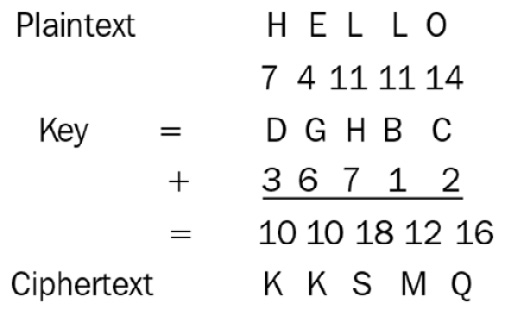Figure 1.10 – Encryption scheme in the Vernam algorithm

So, after transposing the letters, the encryption of `[HELLO]` is `[KKSMQ]`.

You can create an exercise by yourself to decrypt the `[KKSMQ]` ciphertext using the inverse process: applying `f(-K)` to the ciphertext, returning the `HELLO` plaintext.

I would just like to remark that this algorithm is very strong if well implemented, following all the warnings and instructions to avoid a drastic reduction of security. One of the attacks that many algorithms suffer is well known as a ciphertext-only attack. This is successful if the attacker can deduce the plaintext, or even better the key, using the ciphertext or pieces of it. The most common techniques are frequency analysis and traffic analysis.

This algorithm is not vulnerable to ciphertext-only attacks. Moreover, if a piece of a key is known, it will be possible to decipher only the piece corresponding to the related bits. The rest of the ciphertext will be difficult to decrypt if it is long enough. However, the conditions regarding the implementation of this algorithm are very restrictive in order to obtain absolute invulnerability. First of all, the generation of the key has to be completely random. Second, the key and the message have to be of the same length, and third, there is always the problem of the key transmission.

This last problem affects all symmetric algorithms and is basically the problem that pushed cryptographers to invent asymmetric encryption to exchange keys between Alice and Bob (which we will see in the next chapter).

The second problem concerns the length of the key: if the message is too short, for instance, the word `ten`, to indicate the time of a military attack, the attacker could also rely on their good sense or on luck. It doesn't matter if there is a random key for a short message. The message could be decrypted intuitively if the attacker knows the topic of the transmission. On the other hand, if the message is very long, we are forced to use a very long key. In this case, the key will be very expensive to produce and expensive to transmit. Moreover, considering that for every new transmission the key has to be changed, the cost of implementing this cipher for commercial purposes is very high.

This is why, in general, mono-use strings such as this were used for military purposes during the Second World War and after. As I said before, this was the legendary algorithm used for the red line between Washington and Moscow to encrypt communications between the leaders of the US and the USSR during the Cold War.

Finally, we will analyze the implementation of this algorithm. It could be difficult to find a way to generate and transmit a random key, even if the security of the method is very high. In the last section of this book, I will show a new method for the transmission and the implementation of keys using the Vernam cipher combined with other algorithms and methods. This new one-time pad (OTP) system, named Hyper Crypto Satellite, could be used for both the authentication and the encryption of messages. I will also show you the possible vulnerabilities of the system and how to generate a very random key. The method was a candidate at the Satellite International Conference on Space, but at the time I decided not to present it to the public.

# Notes on security and computation

All the algorithms we have seen in this chapter are symmetric. The basic problem that remains unsolved is the transmission of the key. As I've already said, this problem will be overcome by the asymmetric cryptography that we will explore in the next chapter. In this section, we will analyze the computational problem related to the security of cryptographic algorithms generally speaking. Later in the book, we will focus on the security of any algorithm we will analyze.

To make a similitude, we can say that in cryptography the weak link of the chain destroys the entire chain. That is the same problem as using a very strong cryptographic algorithm to protect the data but leaving its password on the computer screen. In other words, a cryptographic algorithm has to be made of a similar grade of security with respect to mathematical problems. To clarify this concept with an example: factorization and discrete logarithm problems hold similar computational characteristics for now; however, if tomorrow one of these problems were solved, then an algorithm that is based on both would be unuseful.

Let's go deeper to analyze some of the principles universally recognized in cryptography. The first statement is Cryptography has to be open source.

With the term open source, I am referring to the algorithm and not, obviously, to the key. In other words, we have to rely on Kerckhoffs' principle, which states the following:

"A cryptosystem should be secure even if everything about the system, except the key, is public knowledge.

Kerckhoffs' principle applies beyond codes and ciphers to security systems in general: every secret creates a potential failure point. Secrecy, in other words, is a prime cause of brittleness—and therefore something likely to make a system prone to catastrophic collapse. Conversely, openness provides ductility."

– Bruce Schneier

In practice, the algorithm that underlies the encryption code has to be known. It's not useful and is also dangerous to rely on the secrecy of the algorithm in order to exchange secret messages. The reason is that, essentially, if an algorithm has to be used by an open community (just like the internet), it is impossible to keep it secret.

The second statement is The security of an algorithm depends largely on its underlying mathematical problem.

As an example, RSA, one of the most famous and most widely used algorithms in the history of cryptography, is supported by the mathematical problem of factorization.

Factorization is essentially the decomposition of a number into its divisors:

`21 = 3 x 7`

It's very easy to find the divisors of `21`, which are `3` and `7`, for small integers, but it is also well known that increasing the number of digits will exponentially increase the problem of factorization.

We will deeply analyze asymmetric algorithms such as RSA in this book, and in particular, in Chapter 3, Asymmetric Encryption, when I will explain asymmetric encryption. But here, it is sufficient to explain why RSA is used to protect financial, intelligence, and other kinds of very sensitive secrets.

The reason is that the mathematical problem underlining RSA (factorization) is still a hard problem to solve for computers of this generation. However, in this introductory section, I can't go deeper into analyzing RSA, so I will limit myself to saying that RSA suffers from not only the problem of factorization as its point of attack, but there is another equally competitive, in computational terms, problem, which is the discrete logarithm problem. Later in the book, we will even analyze both these hard computational problems. Now, we assume (incorrectly, as 99% of cryptographic texts do) that the pillar of security underlying RSA is factorization. In Chapter 6, New Algorithms in Public/Private Key Cryptography, I will show an attack on the RSA algorithm depending on a problem different from factorization. It's the similitude of the weak link of the chain explained at the beginning of this section. If something in an algorithm goes wrong, the underlying security of the algorithm fails.

Anyway, let's see what happens when we attempt to break RSA relying only on the factorization problem, using brute force. In this case, just to give you an idea of the computational power required to decompose an RSA number of 250 digits, factorizing a big semi-prime number is not easy at all if we are dealing with hundreds of digits, or thousands. Just to give you a demonstration, RSA-250 is an 829-bit number composed of 250 decimal digits and is very hard to break with a computer from the current generation.

This integer was factorized in February 2020, with a total computation time of about 2,700 core years with Intel Xeon Gold 6130 at 2.1 GHz. Like many factorization records, this one was performed using a grid of 100 machines and an optimization algorithm that elevated their computation.

The third statement is Practical security is always less secure than theoretical security.

For example, if we analyze the Vernam cipher, we can easily understand how the implementation of this algorithm in practice is very difficult. So, we can say that Vernam is invulnerable but only in theoretical security, not in practical security. A corollary of this assumption is this: implementing an algorithm means putting into practice its theoretical scheme and adding much more complexity to it. So, complexity is the enemy of security. The more complex a system is, the more points of attack can be found.

Another consideration is related to the grade of security of an algorithm. We can better understand this concept by considering Shannon's theory and the concept of perfect secrecy. The definition given by Claude Shannon in 1949 of perfect secrecy is based on statistics and probabilities. However, about the maximum grade of security, Shannon theorized that a ciphertext maintains perfect secrecy if an attacker's knowledge of the content of a message is the same both before and after the adversary inspects the ciphertext, attacking it with unlimited resources. That is, the message gives the adversary precisely no information about the message contents.

To better understand this concept, I invite you to think of different levels or grades of security, in which any of these degrees is secure but with a decreasing gradient. In other words, the highest level is the strongest and the lowest is the weakest but in the middle, there is a zone of an indefinite grade that depends on the technological computational level of the adversary.

It's not important how many degrees are supposed to be secure and how many are not. I think that, essentially, we have to consider what is certainly secure and what is not, but also what can be accepted as secure in a determinate time. With that in mind, let's see the difference between perfect secrecy and secure:

• A cryptosystem could be considered to have perfect secrecy if it satisfies at least two conditions:
• It cannot be broken even if the adversary has unlimited computing power.
• It's not possible to get any information about the message, `[m]`, and the key, `[k]`, by analyzing the cryptogram, `[c]` (that is, Vernam is a theoretically perfect secrecy system but only under determinate conditions).
• A cryptogram is secure even if, theoretically, an adversary can break the cryptosystem (that is, if they had quantum computational power and an algorithm of factorization that runs well) but the underlying mathematical problem is considered at that time very hard to solve. Under some conditions, ciphers can be used (such as RSA, Diffie-Hellmann, and El Gamal) because, based on empirical evidence, factorization and discrete logarithms are still hard problems to solve.

So, the concept of security is dynamic and very fuzzy. What is secure now might not be tomorrow. What will happen to RSA and all of the classical cryptography if quantum computers become effective, or a powerful algorithm is discovered tomorrow that is able to break the factorization problem? We will come back to these questions in Chapter 8, Quantum Cryptography. For now, I can say that most classical cryptography algorithms will be broken by the disruptive computational power of quantum computers, but we don't know yet when this will happen.

Under some conditions, we will see that the quantum exchange of the key can be considered a perfect secrecy system. But it doesn't always work, so it's not currently used. Some OTP systems could now be considered highly secure (maybe semi-perfect secrecy), but everything depends on the practical implementation. Finally, remember an important rule: a weak link in the chain destroys everything.

So, in conclusion, we can note the following:

• Cryptography has to be open source (the algorithms have to be known), except for the key.
• The security of an algorithm depends largely on its underlying mathematical problem.
• Complexity is the enemy of security.
• Security is a dynamic concept: perfect security is only a theoretical issue.

# Summary

In this chapter, we have covered the basic definitions of cryptography; we have refreshed our knowledge of the binary system and ASCII code, and we also explored prime numbers, Fermat's equations, and modular mathematics. Then, we had an overview of classical cryptographic algorithms such as Caesar, Beale, and Vernam.

Finally, in the last section, we analyzed security in a philosophical and technical way, distinguishing the grade of security in cryptography in relation to the grade of complexity.

In the next chapter, we will explore symmetric encryption, where we deep dive into algorithms such as the Data Encryption Standard (DES) and Advanced Encryption Standard (AES) families, and also address some of the issues mentioned in this chapter.

• ##### Massimo Bertaccini

Massimo Bertaccini is a researcher and principal scientist, CEO, and co-founder of Cryptolab Inc.

His career started as a professor of mathematics and statistics. Then, he founded Cryptolab, a start-up in the field of cryptography solutions for cybersecurity. With his team of engineers, he projected and implemented the first search engine in the world that is able to work with encrypted data.

He has obtained several international prizes and awards, including the Silicon Valley inventors award and the Seal of Excellence from the EU.

Currently, he teaches mathematical models at EMUNI University as a contract professor and has published many articles in the field of cryptography, cybersecurity, and blockchain.

Browse publications by this author

### Latest Reviews

(2 reviews total)